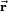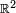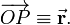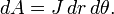# Polar coordinates

For an extension to three dimensions, see spherical polar coordinates.Two dimensional polar coordinates r and θ of vector$\vec{\mathbf{r}}$

In mathematics and physics, polar coordinates are two numbers—a distance and an angle—that specify the position of a point on a plane.

In their classical ("pre-vector") definition, polar coordinates give the position of a point P with respect to a given point O (the pole) and a given line (the polar axis) through O. One real number (r ) gives the distance of P to O and another number (θ) gives the angle of the line OP with the polar axis. Given r and θ, one determines P by constructing a circle of radius r with O as origin, and a line with angle θ measured counterclockwise from the polar axis. The point P is on the intersection of the circle and the line.

In modern vector language one identifies the plane with a real Euclidean space$\mathbb{R}^2$ that has a Cartesian coordinate system. The crossing of the Cartesian axes is on the pole, that is, O is the origin of the Cartesian system and the polar axis is identified with the x-axis of the Cartesian system. The line OP is generated by the vector$\overrightarrow{OP} \equiv \vec{\mathbf{r}}.$

Hence we obtain the figure on the right where$\vec{\mathbf{r}}$ is the position vector of the point P.

## Algebraic definition

The polar coordinates r and θ are related to the Cartesian coordinates x and y through\begin{align} r &= \sqrt{x^2+y^2} \\ x &= r\cos\theta \\ y &= r \sin\theta, \\ \end{align}

so that for r ≠ 0,$\theta = \begin{cases} \arccos(x/r) & \hbox{ if } y \ge 0 \\ 360^0 - \arccos(x/r) & \hbox{ if } y < 0 .\\ \end{cases}$

Bounds on the coordinates are: r ≥ 0 and 0 ≤ θ < 3600. Coordinate lines are: the circle (fixed r, all θ) and a half-line from the origin (fixed direction θ all r). The slope of the half-line is tanθ = y/x.

## Surface element

The infinitesimal surface element in polar coordinates is$dA = J\, dr\,d\theta.$

The Jacobian J is the determinant$J= \frac{\partial(x,y)}{\partial(r,\theta)} = \begin{vmatrix} \cos\theta & -r\sin\theta \\ \sin\theta & r\cos\theta \\ \end{vmatrix} = r \cos^2\theta + r\sin^2\theta = r .$

Example: the area A of a circle of radius R is given by$A = \int_{0}^{2\pi} \int_{0}^R r\, dr\, d\theta = \pi R^2 .$